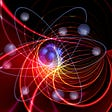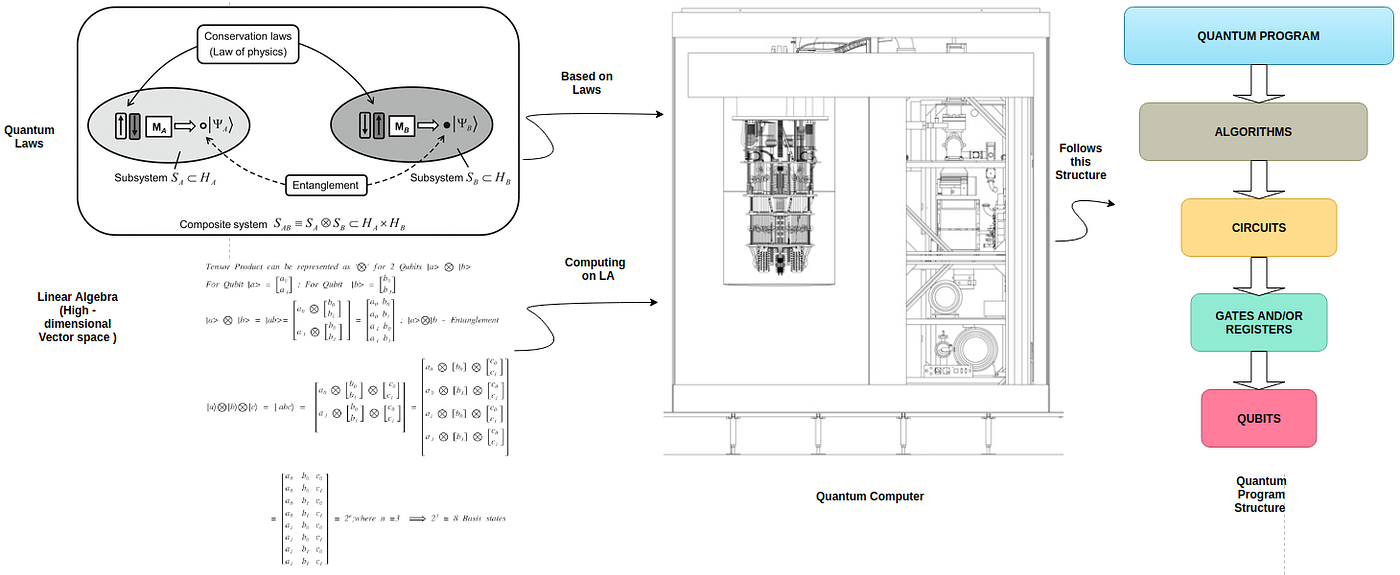Quantum Program requires Quantum Computer ,Quantum Laws and Computational Tool(Linear Algebra)

# Quantum Computing #3-Must have eager to Learn for new Computing

Explored Concepts of Quantum programming in Simple way.

Quantum Computing = Quantum Physics (For Laws) +Linear Algebra (Computation) + Computer Science (Programming)

In this blog, I discuss the concepts of Quantum Programming and elaborate through mathematical perspective and comparing with Classical Computers for easy understanding. The following pic shows the topics that will discuss. Starting from Qubits. It is better to refresh required Linear Algebra.

# Concepts of Quantum Programming

Explaining the concepts in the above diagram in this article except Quantum Algorithms (Algorithms will be in further articles).

Quantum Programming Structure: We will explain this structure from bottom to top.

What is Quantum? The smallest discrete quantity of some physical property that a system can possess.

Introduction of Qubit:

Bit/Qubit: The unit of information is a bit, which can be 0 or 1. Bit is used in Classical Computers. Whereas in Quantum Computing this unit is Quantum Bit(Qubit).

What is Quantum Bit? The unit of information in Quantum Computation is Qubit (or) Quantum Bit. This unit is a superposition of ‘0’ or ‘1’.

In classical computing, bits are the fundamental object of information. The Quantum equivalent of a bit is called “Qubit” or “Quantum Bit”.

Classical Computing bits can take 0-Zero (or) 1-One.

Quantum Computing bits can take 0-Zero (or) 1-One (or) both (0-1 )at the same time.

Quantum Properties: To manipulate the state of a Qubit using ‘3’ properties , they are Superposition, Entanglement and Interference.

Superposition: It refers to a linear combination of states we would ordinarily describe independently. For Example: If you play ‘2’ Musical Notes at, what you will hear is a Superposition of 2 Notes. |St> = a |0> + b |1>.

Entanglement: Entangled particles (Bodies) behave together as a system in ways that cannot be possible in classical systems.

Interference: A Quantum System can exhibit interference effects during the course of evolution.

Basis State/Vector: Consider a System with 2 basis states, call them 0 and 1 (only single digit) in Classical Computers and vectors in Quantum Computing as |0> & |1> (vector).

Vectors can represented in Dirac Notation is as follows:

A single Qubit can be in any superposition, it can take |0> or |1> or both |0>+|1> with their probabilities.

Quantum State: A state can be in ‘0’ or ‘1’ in Classical Computers, whereas in Quantum Systems it is a vector of basis states. Any Quantum State can be held by a Qubit of any two-dimensional column vector of real or complex numbers with their norm 1.

A single Qubit represented in 2D vector and their norm is ‘1’.

Number Dimensions or Basis states for Qubits :

Question : if there are ’n’ qubits in the system then how many basis states or dimension in the system.

# Dirac Notation:

Dirac presented an alternative formulation/notation of Quantum Mechanics by means of Bra-Ket Notation.

Consider a Quantum System with 2 basis states (0 &1) can be represented as |0> & |1>. Dirac Representation in Quantum Systems is defined as follows.

In this notation Ket is |0> Column vector, <0| Bra is Row vector. It is Bra-Ket for Row and Column Vector representation.

Bra–Ket notation is a common notation for Quantum States, i.e. vectors in a complex Hilbert Space. It is concise and convenient way to describe Quantum States. It is the standard notation in Quantum Mechanics/Theory. In this notation have shown how Linear Algebra involved.

A Single Qubit can be in any superposition & represented as a linear combination of Basis States |0>, |1>, often called “Superposition”.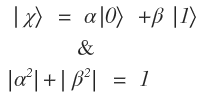Qubit in Superposition and their amplitudes square must be 1 (Probability Axiom applied)

Quantum Statevector: Statevector holds the Amplitudes or Probabilities for the states. We are operating on Amplitudes or probabilities i.e Statevectors.

A single Qubit “lives” in the vector space C — complex numbers.

Qubit Measurement: Simple rule for measurement. To find the Probability of measuring a state |st>(Qubit) in the state |x>.

For example measuring state |0> in the Qubit q.

Visualization of a Qubit State: “Bloch Vector is a visualization Tool”. Qubits may also be pictured in 3D using the Bloch Sphere representation.

Bloch Sphere describing a single-qubit quantum state (2D Complex Vector) as 3D Real valued Vector.

Bloch sphere can be visualize is as follows:

Arrow represents the direction in which quantum state vector is pointing and each transformation of the arrow can be though of as a rotation about one of the cardinal axes.

Note that In Multi-Qubit representation Bloche Sphere breaks down.

Multiple Qubits

As of now, we considered only one Qubit, we move on to Multi-Qubits. Let us see how Qubits interact with each other.

The Quantum Property Entanglement comes into picture while communicate between Qubits.

Representing Multi-Qubit States: If we have 2 different Qubits & their collective states can be described using Tensor Product. The following Notation describe Tensor Product between 2 Qubits.

Number of Basis states for 2-Qubit system has 4 basis states:

# Quantum Computing Programming Structure

Basic Structure of Quantum Program:

The structure explains that Program work on Algorithm, Algorithm works on Circuits -> Circuits works on Registers and Gates -> Gates works on works on Qubits (Single or Multiple).

Representation of Qubit Operations,Gates in terms of Linear Algebra Objects (Vectors, Matrices and Tensors) & LA Stuff in the below diagram.

Qubit : The Unit of information in Quantum Computation is Qubit (or) Quantum Bit. There are 2 types of Qubits are Single Qubit &Multiple Qubit.

For detailed information on Qubit see section A Brief Introduction of Qubit: above.

Single Qubit: Only one Qubit operating at a time.

Multiple Qubit: More than one Qubit operating at a time.

# Quantum Gates:

Quantum Gate: A unitary that acts on a small number of Qubits is called a Gate. In Classical computers gates are Logical (AND, OR & NOT). Operations can be done in Gates. Quantum Gates are represented in the form of Matrices.

Brief explanation of Gates below.

Types of Qubits ,Gates and their Operations:

Single Qubit Gates are Pauli Matrices: Pauli-X, Pauli-Y, Pauli-Z Gates, Hadamard Gate, R-phi Gate, I, S, T Gate, U3 Gate.

Pauli-X Gate: X-gate is NOT Gate in Classical Computers.

Pauli-Y & Z Gates:

Hadamard Gate: It is basically Quantum Gate. It has the matrix:

Note : X Gate ( NOT Gate) can be represented as X = HZH

I,S and T-Gates: I-Gate known as Id-Gate or Identity Gate. It does nothing,it is simply a gate.

S-Gate: This is an R-phi gate with phi = pi/2, it can be shown in the following

T-gate: T-gate is very commonly used gate, it is an R-phi gate with phi = pi/4

General U-gates:

I,Z,S & T-gates were all special cases of the more general R-phi Gate. Like that U3-Gate is the most general of all single-qubit quantum gates. It is a parameterised gate of the form:

Two Qubit Gates — Controlled Not(CNOT) Gate.

In Quantum Circuit language we have two wires, representing two Qubits, and following notation to represent the CNOT gate:

In the Circuit wire with the small, filled dot on it is Called the Control Qubit and the wire with the larger, unfilled circle on it is called the “Target” Qubit.

As we know for 2-Qubits we have four possible states of a two-bit system :

|00>,|01>,|10> and |11>. We can also have superpositions (i.e., linear combinations)of them:

Now , the CNOT Gate is, much like the Quantum NOT Gate. If the Control Qubit is set to 1, then it flips the (i.e.,NOTs) the target Qubit, otherwise it does nothing. In out 4 Basis states it will effect |10> and |11> basis states.

After CNOT on 4 Basis states the result is:

If you summing up all four of the equations above in a single equation (i.e., CNOT on 2-Qubit 4 Basis States) and assume x and y are classical bits i.e., 0 or 1. Then the operation tells us that it is XOR Operation on 2 classical bits. i.e.,

CNOT Gate makes clear that the CNOT leaves the control qubit x alone, but flips the target qubit y if x is set to 1.

Note that it acts linearly on superposition of computational basis states, same as expect for a quantum gate.

Three Qubit Gates — Tiffoli Gate (Controlled-Controlled-Not-Gate)

CNOT Gate not only applied 2-Qubits computations. It is applicable to more Qubits. For 3-Qubits basis states are |000>,|001>,|010>,|011>,|100>,

|101>,|110>,|111>.

Here first bit ‘x’ is not changed at all, since it is not involved in the CNOT.

# Quantum Circuits:

Quantum Circuit: A Quantum Circuit (Quantum Network or Quantum Gate Array) generalizes the idea of classical computer Circuit. Quantum Circuit is a model for Quantum Computation is a sequence of Quantum Gates.

Simply Circuit will execute Gate Operations (All Gates in Matrix form as explained previously)

Quantum Register: A number of Qubits taken together is a Qubit Register. Quantum Computers perform calculations by manipulating Qubits within a Quantum Register.

Quantum Register for 3 Qubits will be taken is as follows. As per the number of basis states formula totally we have 8 basis states for 3 Qubits. Following pic describes this.

For example : Let’s do an X-gate on a |0> qubit ; Implementing Qiskit Framework:

qc = QuantumCircuit(1) — Build Circuit

qc.x(0) — Add Gate to Circuit

qc.draw(‘mpl’) — Draw the Circuit

The Quantum Circuit is :

Another Example for H-Gate, CNOT-Gate, CNOT-Gate in a Circuit & the circuit execution is as follows

Add a H gate on qubit 0,

Code :circ = QuantumCircuit(3),circ.h(0),

Add a CX (CNOT) gate on control qubit 0 and target qubit 1,

Code: circ.cx(0, 1)

Add a CX (CNOT) gate on control qubit 0 and target qubit 2,

Code : circ.cx(0, 2)

The Circuits is :

Quantum Algorithms:

Quantum Algorithms can be broadly classified in the following categories.

# Quantum Program:

We use these algorithms in our Quantum Program based on the requirement. Quantum Machine Learning Algorithms come into Hybrid Quantum Classical Algorithms category.

For the sake of Quantum Program, I chose Simon’s Algorithm for explaining the steps of Quantum Program. Algorithms explained in further articles.

Please note that Circuit implements the Algorithm, below shows Quantum Circuit for Simon’ s Algorithm for 2 Qubits.

In Simple terms Quantum Program’s Pseudo Code is as follows:

1. Create Quantum Registers for ’n’ Qubits
2. Create Quantum Circuit and add Quantum Registers to Circuit
3. Apply the gates to Circuits to work on Qubits
4. Do any further processing on Qubits
5. Visualize the Qubits
6. Measure the Qubits
7. Draw the Circuit

Apart from these steps there is a lot of work and components involve to execute Program behind the scenes.

The Quantum Program for Simon’s Algorithm is

`b = '110'n = len(b)simon_circuit = QuantumCircuit(n*2, n)# Apply Hadamard gates before querying the oraclesimon_circuit.h(range(n))        # Apply barrier for visual separationsimon_circuit.barrier()simon_circuit += simon_oracle(b)# Apply barrier for visual separationsimon_circuit.barrier()# Apply Hadamard gates to the input registersimon_circuit.h(range(n))# Measure qubitssimon_circuit.measure(range(n), range(n))simon_circuit.draw()`

Opensource Quantum Computing full-stack Libraries:

Cirq — from Google ,Q# — From Microsoft , it supports 3 languages Csharp,Qsharp and python,Qiskit — From IBM ,Qiskit-JS IBM’s quantum information software kit for JavaScript, Strawberry Fields, XACC, Forest , Ocean, Project Q , TensorFlow-Quantum, etc.,

Quantum Simulation:

Quantum Simulators allows us to simulate quantum behaviours using classical hardware. We can test and optimize any circuits and solutions on IBM’s high-performance simulators locally or on the cloud, and compare them to real quantum devices in a streamlined environment.

Some common simulators for quantum computing are BLACK-STONE, QSimulator.jl, Cliffords.jl, Liquid, QCL,etc.,

Conclusion:

Quantum Mechanics/Theory developed in a span of 25 years, for understanding it may take some time than understanding classical computation.

Mostly working in Linear Algebra , but notation is in Dirac Notation — Notation is different , not concept. Adding layer by layer on Quantum Theory becomes a computational model.

Thanks for reading my article , drop a note for your comments, feedback and mistakes, if any.

# References :

Quantum Computation and Quantum Information 10th Edition — Cambridge University Press — By Michael A.Nielsen & Issac L.Chuang

Oxford University Press — An Introduction to Quantum computing By Phillip Kaye, Raymond Laflamme, Michele Mosca

--

--

--

## More from Shafi

Data Architect, Researcher in AI & Quantum Computing. Passionate in Autonomous.

Love podcasts or audiobooks? Learn on the go with our new app.

## Famous Modern Math Problems: The Erdos-Turan Conjecture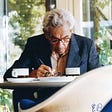## 1–2–3 Pattern: Easy to Hard## The Least Efficient Way to Teach Math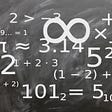## Creating Maximally Entangled State Using Controlled-Y Gate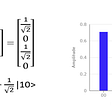## Math clarification for learning NLP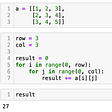## Famous Modern Math Problems: The Square Peg Problem## Shafi

Data Architect, Researcher in AI & Quantum Computing. Passionate in Autonomous.

## What I Learned From Fire Opal…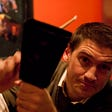## Q-Intro: Grover Iterator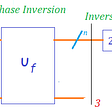## The Road to a 50-Qubit Quantum Computer and Quantum Supremacy: Challenges & Future Applications